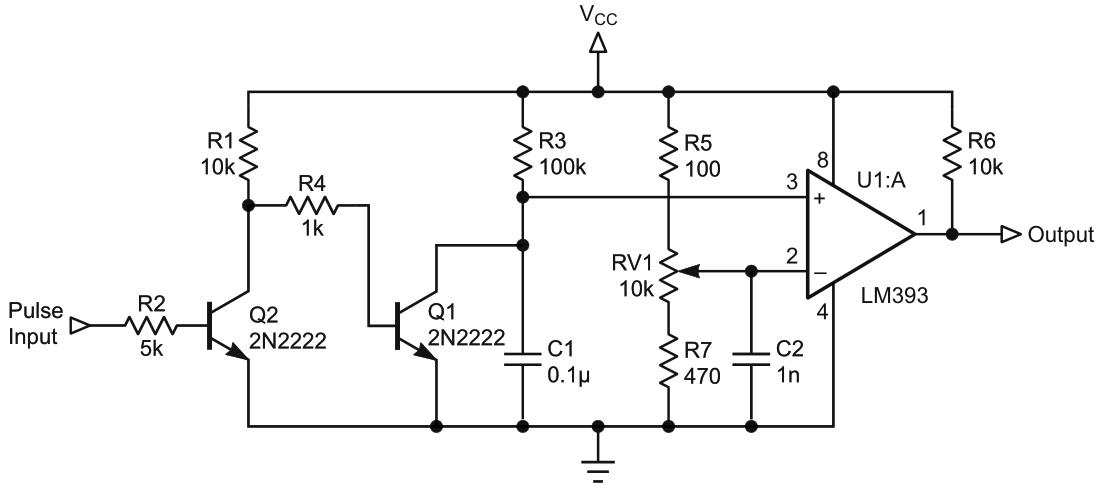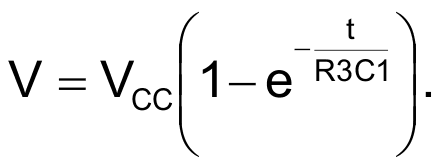# A long pulse detector circuit

## Texas Instruments LM393

There are several applications in which pulses longer than the set width are to be detected, ignoring the other short pulses. We are familiar with the missing pulse detector circuit, which detects the occurrence of a missing pulse in a pulse train where the pulses are of equal duration. But this circuit detects the long pulses in a pulse train whose pulses need not be of equal duration. The circuit in Figure 1 outputs the pulses which are longer than the set width. This output can be counted or taken for further action as needed.Figure 1. Circuit detects the long pulse whose width is decided by RV1’s setting. The long pulse alone will be output while other short pulses are not. See text for RV1’s potentiometer setting.

During OFF durations of the pulse train, capacitor C1 is shorted by Q1 making the capacitor voltage zero. Comparator LM393’s output becomes zero. During the ON duration of the pulse train, capacitor C1 charges, whose instantaneous voltage is given by:Calculate the value of V corresponding to the long pulse duration. Then set the potentiometer voltage at the viper to this value. For shorter pulses, the capacitor voltage will be < V, hence the comparator outputs zero. For a long pulse, the capacitor charge voltage will be > V, hence comparator outputs a high. Thus, we get pulses at the comparator output whenever input pulse widths exceed the required pulse width, as set by the potentiometer set voltage V.

Figure 1’s circuit values are designed to track pulses with a duration longer than 2 ms. As per the above charge equation, V comes to 0.9 V for a pulse width of 2 ms. The potentiometer VR1 is set at 0.9 V. For longer pulses, the capacitor charge voltage will be more than 0.9 V and the comparator will output high, indicating the arrival of a long pulse. Pulses shorter than 2 ms will produce voltage less than 0.9 V and the output will be zero. Thus, short duration pulses are neglected.

EDN